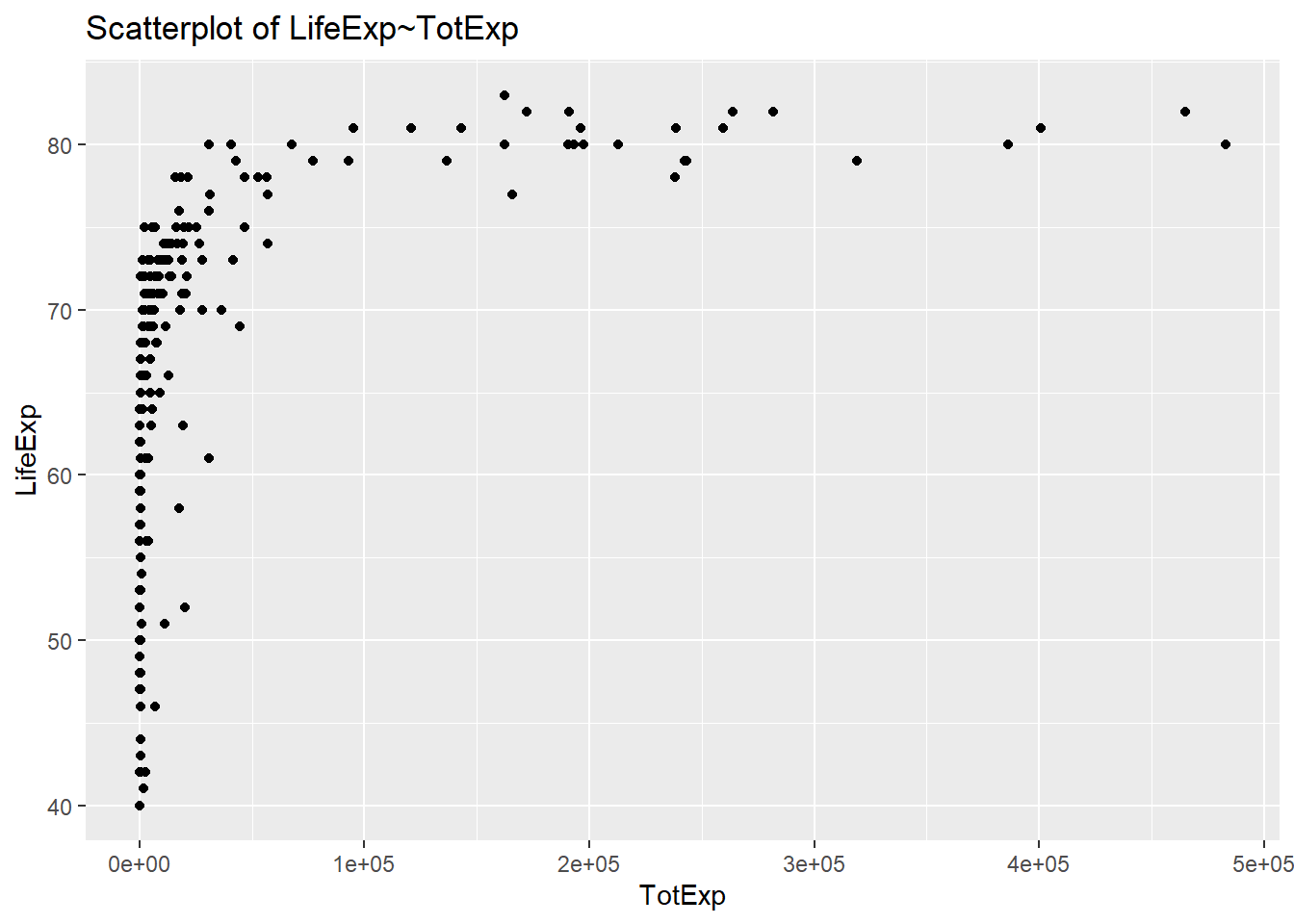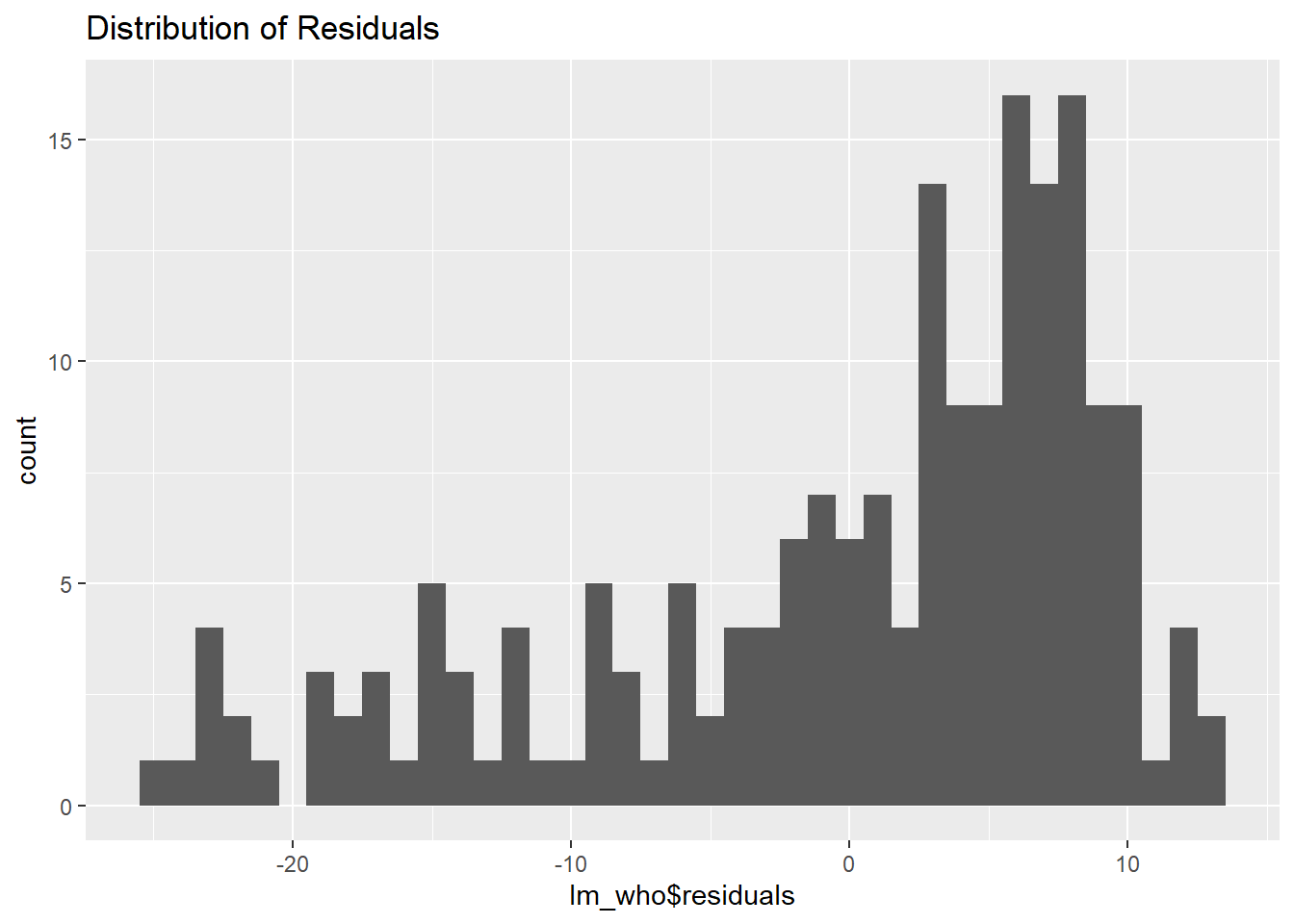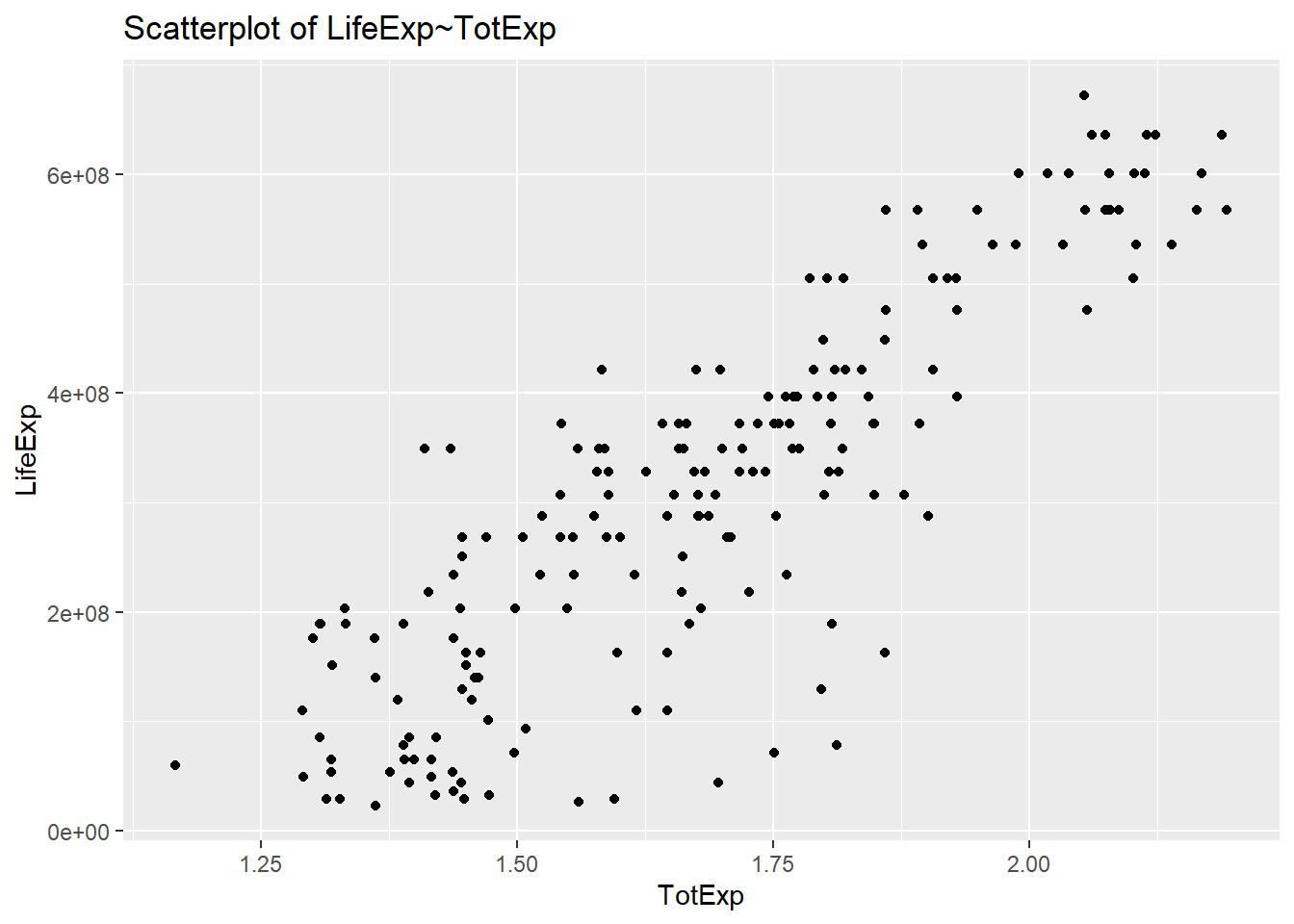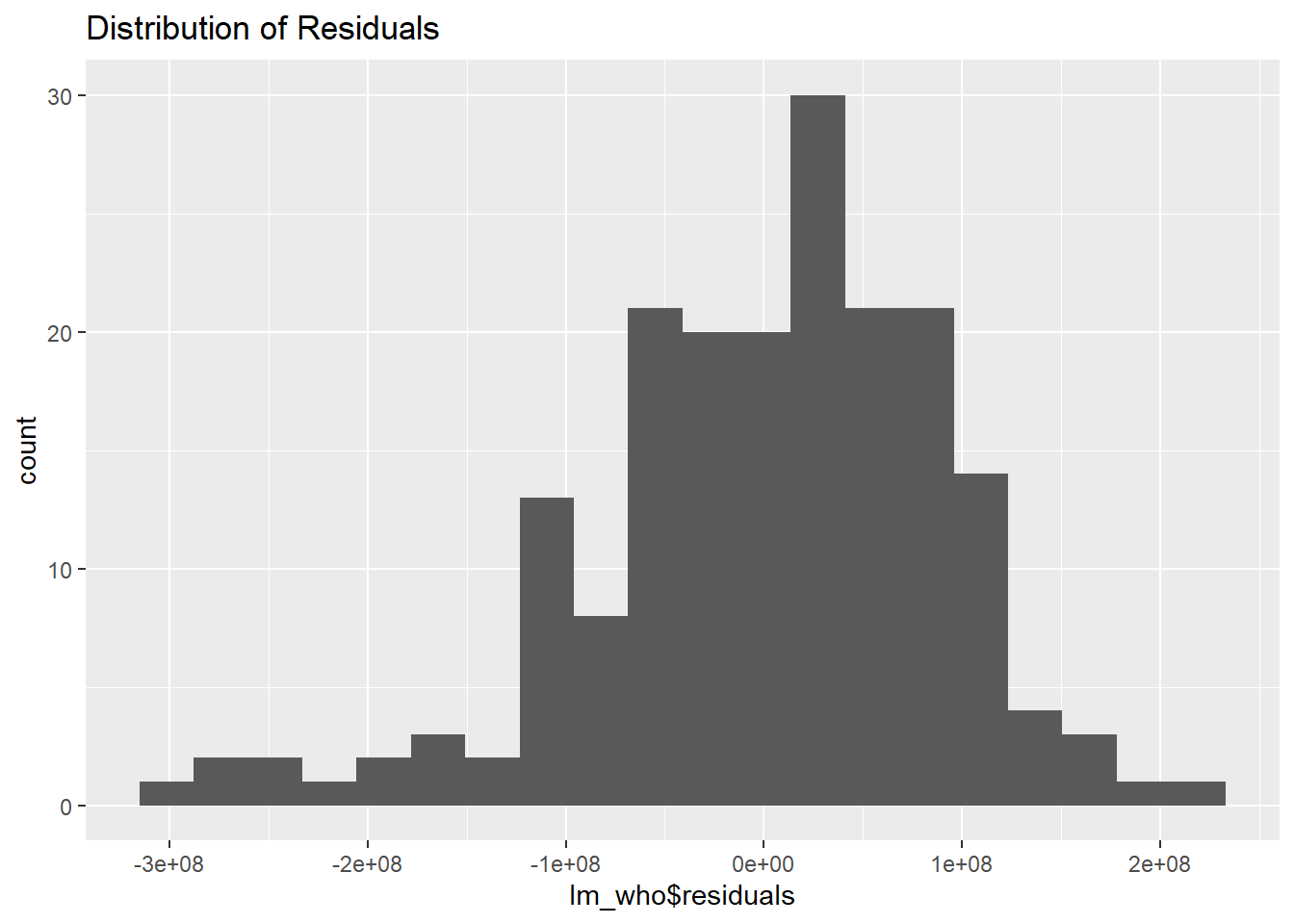# Assignment 12

## Dataset

The attached who.csv dataset contains real-world data from 2008. The variables included follow.

• Country: name of the country

• LifeExp: average life expectancy for the country in years

• InfantSurvival: proportion of those surviving to one year or more

• Under5Survival: proportion of those surviving to five years or more

• TBFree: proportion of the population without TB.

• PropMD: proportion of the population who are MDs

• PropRN: proportion of the population who are RNs

• PersExp: mean personal expenditures on healthcare in US dollars at average exchange rate

• GovtExp: mean government expenditures per capita on healthcare, US dollars at average exchange rate

• TotExp: sum of personal and government expenditures.

who <- read.csv("https://raw.githubusercontent.com/mkivenson/Computational-Mathematics/master/Assignments/Assignment%2012/who.csv")

head(who)
##               Country LifeExp InfantSurvival Under5Survival  TBFree
## 1         Afghanistan      42          0.835          0.743 0.99769
## 2             Albania      71          0.985          0.983 0.99974
## 3             Algeria      71          0.967          0.962 0.99944
## 4             Andorra      82          0.997          0.996 0.99983
## 5              Angola      41          0.846          0.740 0.99656
## 6 Antigua and Barbuda      73          0.990          0.989 0.99991
##        PropMD      PropRN PersExp GovtExp TotExp
## 1 0.000228841 0.000572294      20      92    112
## 2 0.001143127 0.004614439     169    3128   3297
## 3 0.001060478 0.002091362     108    5184   5292
## 4 0.003297297 0.003500000    2589  169725 172314
## 5 0.000070400 0.001146162      36    1620   1656
## 6 0.000142857 0.002773810     503   12543  13046

## Step 1

Provide a scatterplot of LifeExp~TotExp, and run simple linear regression. Do not transform the variables. Provide and interpret the F statistics, R^2, standard error,and p-values only. Discuss whether the assumptions of simple linear regression met.

#### Scatterplot

ggplot(who, aes(TotExp, LifeExp)) + geom_point() +
ggtitle("Scatterplot of LifeExp~TotExp")#### Linear regression

attach(who)
lm_who <- lm(LifeExp~TotExp)
summary(lm_who)
##
## Call:
## lm(formula = LifeExp ~ TotExp)
##
## Residuals:
##     Min      1Q  Median      3Q     Max
## -24.764  -4.778   3.154   7.116  13.292
##
## Coefficients:
##              Estimate Std. Error t value Pr(>|t|)
## (Intercept) 6.475e+01  7.535e-01  85.933  < 2e-16 ***
## TotExp      6.297e-05  7.795e-06   8.079 7.71e-14 ***
## ---
## Signif. codes:  0 '***' 0.001 '**' 0.01 '*' 0.05 '.' 0.1 ' ' 1
##
## Residual standard error: 9.371 on 188 degrees of freedom
## Multiple R-squared:  0.2577, Adjusted R-squared:  0.2537
## F-statistic: 65.26 on 1 and 188 DF,  p-value: 7.714e-14

#### Assumptions

• Linear relationship - Not met, the scatterplot is not linear.
• Normally distributed Residuals - Not met, the residuals are skewed.
ggplot(lm_who, aes(x=lm_who$residuals)) + geom_histogram(binwidth=1) + ggtitle("Distribution of Residuals")• Independence of the observations - population health a country must be independent of population health of other countries (assume true for this exercise?) • Homoscedasticity - Variance appears constant #### Results • The F-statistic of 65.26 is the overall significance of the regression model. • The R^2 of 0.2577 means that the fit of the model is subpar • The Standard Error of 9.371 is the amount which the estimated life expectance varies from the actual value. • The p-value of 7.71e-14 indicates the relationship between LifeExp and TotExp is significant ## Step 2 Raise life expectancy to the 4.6 power (i.e., LifeExp^4.6). Raise total expenditures to the 0.06 power (nearly a log transform, TotExp^.06). Plot LifeExp^4.6 as a function of TotExp^.06, and r re-run the simple regression model using the transformed variables. Provide and interpret the F statistics, R^2, standard error, and p-values. Which model is “better?” #### Transformations who$LifeExp <- who$LifeExp^4.6 who$TotExp <- who$TotExp^.06 #### Linear regression ggplot(who, aes(TotExp, LifeExp)) + geom_point() + ggtitle("Scatterplot of LifeExp~TotExp")#### Scatterplot attach(who) ## The following objects are masked from who (pos = 3): ## ## Country, GovtExp, InfantSurvival, LifeExp, PersExp, PropMD, ## PropRN, TBFree, TotExp, Under5Survival lm_who <- lm(LifeExp~TotExp) summary(lm_who) ## ## Call: ## lm(formula = LifeExp ~ TotExp) ## ## Residuals: ## Min 1Q Median 3Q Max ## -308616089 -53978977 13697187 59139231 211951764 ## ## Coefficients: ## Estimate Std. Error t value Pr(>|t|) ## (Intercept) -736527910 46817945 -15.73 <2e-16 *** ## TotExp 620060216 27518940 22.53 <2e-16 *** ## --- ## Signif. codes: 0 '***' 0.001 '**' 0.01 '*' 0.05 '.' 0.1 ' ' 1 ## ## Residual standard error: 90490000 on 188 degrees of freedom ## Multiple R-squared: 0.7298, Adjusted R-squared: 0.7283 ## F-statistic: 507.7 on 1 and 188 DF, p-value: < 2.2e-16 #### Assumptions • Linear relationship - Met, the adjusted relationship is moderately strong, positive, and linear. • Normally distributed Residuals - Met, the residual histogram is normal. ggplot(lm_who, aes(x=lm_who$residuals)) +
geom_histogram(bins = 20) +
ggtitle("Distribution of Residuals")• Independence of the observations - population health a country must be independent of population health of other countries (assume true for this exercise?)
• Homoscedasticity - Variance in the scatterplot appears constant

#### Results

• The F-statistic of 507.7 is the overall significance of the regression model.
• The R^2 of 0.7298 means that the model fits the data well
• The Standard Error of 90490000 is the amount which the estimated life expectance^4.6 varies from the actual value.
• The p-value of <2e-16 indicates the relationship between LifeExp and TotExp is significant

## Step 3

Using the results from 3, forecast life expectancy when TotExp^.06 = 1.5. Then forecast life expectancy when TotExp^.06=2.5.

#### Model

$LifeExp^{4.6} = -736527910 + 620060216 * TotExp^.06$

exp_model <- function(x){
y = -736527910 + (620060216 * x)
return(y)
}

#### TotExp^.06 = 1.5

exp_model(1.5)
##  193562414

#### TotExp^.06 = 2.5

exp_model(2.5)
##  813622630

## Step 4

Build the following multiple regression model and interpret the F Statistics, R^2, standard error, and p-values. How good is the model? LifeExp = b0+b1 x PropMd + b2 x TotExp +b3 x PropMD x TotExp

#### Multiple regression

attach(who)
## The following objects are masked from who (pos = 3):
##
##     Country, GovtExp, InfantSurvival, LifeExp, PersExp, PropMD,
##     PropRN, TBFree, TotExp, Under5Survival
## The following objects are masked from who (pos = 4):
##
##     Country, GovtExp, InfantSurvival, LifeExp, PersExp, PropMD,
##     PropRN, TBFree, TotExp, Under5Survival
mult_lm_who <- lm(LifeExp ~ +
PropMD +
TotExp +
(PropMD * TotExp))
summary(mult_lm_who)
##
## Call:
## lm(formula = LifeExp ~ +PropMD + TotExp + (PropMD * TotExp))
##
## Residuals:
##        Min         1Q     Median         3Q        Max
## -296470018  -47729263   12183210   60285515  212311883
##
## Coefficients:
##                 Estimate Std. Error t value Pr(>|t|)
## (Intercept)   -7.244e+08  5.083e+07 -14.253   <2e-16 ***
## PropMD         4.727e+10  2.258e+10   2.094   0.0376 *
## TotExp         6.048e+08  3.023e+07  20.005   <2e-16 ***
## PropMD:TotExp -2.121e+10  1.131e+10  -1.876   0.0622 .
## ---
## Signif. codes:  0 '***' 0.001 '**' 0.01 '*' 0.05 '.' 0.1 ' ' 1
##
## Residual standard error: 88520000 on 186 degrees of freedom
## Multiple R-squared:  0.7441, Adjusted R-squared:   0.74
## F-statistic: 180.3 on 3 and 186 DF,  p-value: < 2.2e-16

#### Results

• The F-statistic of 180.3 is the overall significance of the regression model.
• The R^2 of 0.7441 means that the model fits the data well
• The Standard Error of 88520000 is the amount which the estimated life expectance^4.6 varies from the actual value.
• The p-value of <2e-16 indicates the linear model is significant

## Step 5

Forecast LifeExp when PropMD=.03 and TotExp = 14. Does this forecast seem realistic? Why or why not?

#### Prediction

mult_exp_model <- function(a,b){
y = -724400000 +
(47270000000 * a) +
(604800000 * b) +
(-21210000000 * a * b)
return(y)
}
(mult_exp_model(0.03, 14))^(1/4.6)
##  67.08931

This result seems reasonable because we used the adjusted exponential model. I will check results with the original dataset as well.

Here, I re-did the multiple linear regression with the original dataset (without adjusting LifeExp and TotExp).

who <- read.csv("https://raw.githubusercontent.com/mkivenson/Computational-Mathematics/master/Assignments/Assignment%2012/who.csv")

#### Multiple regression

attach(who)
## The following objects are masked from who (pos = 3):
##
##     Country, GovtExp, InfantSurvival, LifeExp, PersExp, PropMD,
##     PropRN, TBFree, TotExp, Under5Survival
## The following objects are masked from who (pos = 4):
##
##     Country, GovtExp, InfantSurvival, LifeExp, PersExp, PropMD,
##     PropRN, TBFree, TotExp, Under5Survival
## The following objects are masked from who (pos = 5):
##
##     Country, GovtExp, InfantSurvival, LifeExp, PersExp, PropMD,
##     PropRN, TBFree, TotExp, Under5Survival
mult_lm_who <- lm(LifeExp ~ +
PropMD +
TotExp +
(PropMD * TotExp))
summary(mult_lm_who)
##
## Call:
## lm(formula = LifeExp ~ +PropMD + TotExp + (PropMD * TotExp))
##
## Residuals:
##     Min      1Q  Median      3Q     Max
## -27.320  -4.132   2.098   6.540  13.074
##
## Coefficients:
##                 Estimate Std. Error t value Pr(>|t|)
## (Intercept)    6.277e+01  7.956e-01  78.899  < 2e-16 ***
## PropMD         1.497e+03  2.788e+02   5.371 2.32e-07 ***
## TotExp         7.233e-05  8.982e-06   8.053 9.39e-14 ***
## PropMD:TotExp -6.026e-03  1.472e-03  -4.093 6.35e-05 ***
## ---
## Signif. codes:  0 '***' 0.001 '**' 0.01 '*' 0.05 '.' 0.1 ' ' 1
##
## Residual standard error: 8.765 on 186 degrees of freedom
## Multiple R-squared:  0.3574, Adjusted R-squared:  0.3471
## F-statistic: 34.49 on 3 and 186 DF,  p-value: < 2.2e-16

#### Results

This model is much less accurate than the adjusted version, with an R^2 of 0.3574.

#### Prediction

The prediction of 107.68 years does not seem accurate, suggesting that this model is not accurate.

mult_exp_model <- function(a,b){
y = 62.77 +
(1497 * a) +
(.0000723300 * b) +
(-.006026 * a * b)
return(y)
}
mult_exp_model(0.03, 14)
##  107.6785